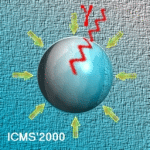# ICMS'2000

The International Conference on Multiphase Systems, ICMS'2000
Ufa, RUSSIA, June 15-17, 2000

Stability and Dynamics of the Core-Annular Flow in a Periodically Constricted Tube

Kouris Ch., Tsamopoulos J.

Laboratory of Computational Fluid Dynamics, Department of Chemical Engineering, University of Patras, Patras, GREECE

Both the stability and the time evolution of the concentric two-phase flow of two immiscible fluids in a tube of sinusoidally varying cross section are studied. The model equations depend on six dimensionless parameters: the Reynolds, Weber and Froude numbers and the ratios of density, viscosity and volume of the two fluids. Two more dimensionless numbers describe the shape of the solid wall: the constriction ratio and the ratio of its maximum radius to its period. Our both stability calculations and dynamic simulations are performed by approximating every dependent variable using Chebyshev polynomials in the radial and Fourier modes in the axial direction.

Arnoldi's algorithm is used to compute the unstable eigenvalues of the linearized equations of motion of the two-phase flow in both two- and three- dimensional disturbances. Extensive calculations for the dependence of the neutral stability boundaries on the various parameters are performed. Three-dimensional instabilities do not arise before the two-dimensional ones. In most cases that the steady solution is found to become unstable it does so through a Hopf bifurcation.

The time integration of the non-linear axisymmetric set of equations is performed using the 2nd order Adams-Moulton method. The time evolution of the core-annular flow (CAF), for such values of the dimensionless numbers that the steady solution becomes unstable, reveals that indeed travelling waves bifurcate from the perfect CAF which although grow exponentially at first, saturate as a result of nonlinear mechanisms.